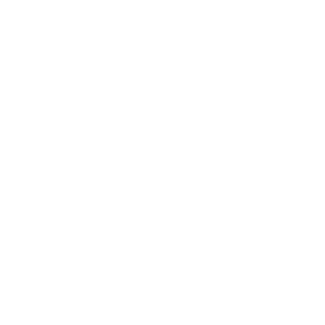# Web Puzzles

Showing posts with label play logical games. Show all posts

## Deneb

She was an absent minded one, forever seeing things that interest her intellilect and hold her in thought for hours on end. On this particular time, she saw a curious symbol, her mental processes setting formulating a problem to solve.Starting at any point, how can you draw the whole symbol by tracing along the lines and never tracing along the same line twice?

### Deneb Puzzle Solution## FrogsFour ladies are facing each other. Each one of them speaks twice. One of those statements is truth, the other is a lie.

Amanda:
"I am a frog but I look like a princess."
"There are at least three frogs between the four of us."

Barbara:
"I'm a princess."
"Corinna always lies."

Corinna:
"There is only one frog here."
"I'm a frog that looks like a princess."

Deborah:
"Two of us are frogs."
"I'm a princess."

Are there any frogs? If so, what are their names

### Frogs Puzzle Solution

The only frog is Amanda. The table below shows, on the left, all possible combinations of frogs (X stands for frog, - stands for non-frog), while on the right pane there is the control of the truthfullness (T for true, F for false) of each given statement, for each combination of frog population. The only combination where every girl tells one true statement and one false one, is the emphasised row. Hence, there's only one frog, and that's Amanda.
Possible Frogs Statement
A B C D A B C D
- - - - F F T F F F F T
- - - X F F T F T F F F
- - X - F F T F T T F T
- - X X F F T T F T T F
- X - - F F F F T F F T
- X - X F F F F F F T F
- X X - F F F T F T T T
- X X X F T F T F T F F
X - - - T F T F T F F T
X - - X T F T F F F T F
X - X - T F T T F T T T
X - X X T T T T F T F F
X X - - T F F F F F T T
X X - X T T F F F F F F
X X X - T T F T F T F T
X X X X T T F T F T F F